# ECDSA Algorithm and Elliptic CurvesIn this article, we learn about the ECDSA algorithm and how it is used in the Bitcoin and Ethereum networks to secure transactions and funds.

1. Introduction.
2. The ECDSA Algorithm.
3. The Elliptic curve.
4. Mathematics involved with Elliptic curves.
5. Summary.
6. References.

## Introduction

The ECDSA (Elliptic Curve Digital Signature algorithm) is a cryptographic algorithm used in the Bitcoin and Ethereum Blockchains. In blockchains, a combination of hashing and asymmetric cryptography is used to secure transactions and messages.

A public key is generated together with the private key as a key pair. The public key is public knowledge but under no circumstances is the private key to be disclosed.

Digital signatures are also used to validate transactions between blockchain users.

## The ECDSA Algorithm.

The ECDSA algorithm consists of two algorithms, the signing and verification that both use important variables to acquire a signature and then reverse the process of getting a message given a signature. This is what is used to control the ownership of coins on the bitcoin blockchain.

The following is an image of an Elliptic Curve.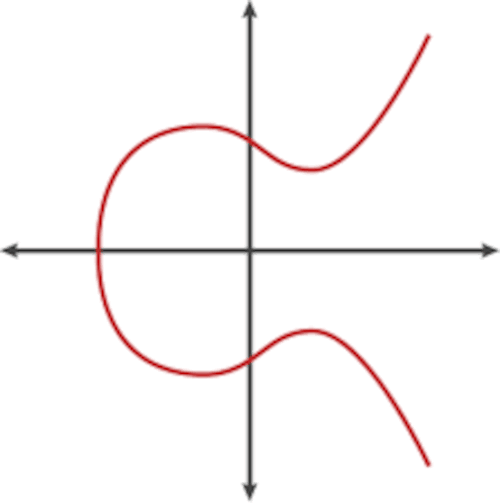Elliptic curve cryptography is public-key cryptography based on the algebraic structure of elliptic curves over finite fields. The security of elliptic cryptography all depends on the ability to be able to compute point multiplication and the inability to compute the multiplicand given the original and product points. More on this can be found in the links provided in the references section.

Public keys and digital signatures are points on the elliptic curve. If both points are generated from one private key, there will be a geometric connection between the points proving that the creator of the signature also owns the public key. Among the many different elliptic curves, secp256k1 is the one chosen for Bitcoin.

By using digital signatures users can generate a key pair of public and private keys, then use the private key to generate a digital signature proving that they own the public key. This is demonstrated below;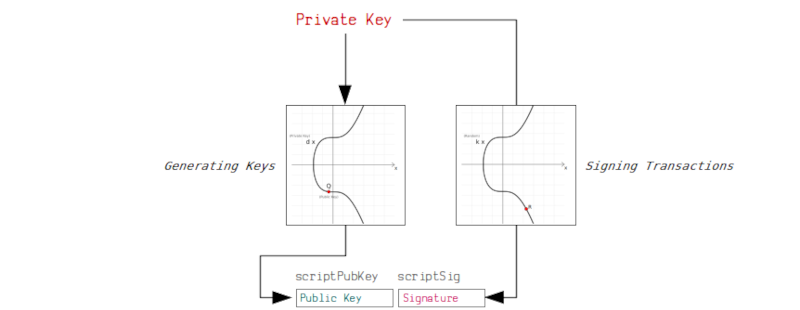On the blockchain, all nodes recognize that a certain participant is the rightful owner of certain coins. In addition to this, the public-private key pair is also essential to be able to access and transfer funds. This is because a valid signature is needed for a transaction, a signature generated by a private key for the public key that was used to generate the signature.

In this article, we will learn about elliptic curves, elliptic curve cryptography, and the mathematics that goes into this algorithm. We will also implement the functions using the Python programming language.

## The Elliptic curve.

Let's define an elliptic curve as a set of points that are described by the following equation;

y^2 = x^3 + ax + b mod p

Among the various types of elliptic curves, secp256k1 was chosen for the implementation of cryptography in Bitcoin.

• secp256k1 is broken down as follows;
• sec - Standard for Efficient Cryptography.
• p - prime(a prime number for creating a finite field).
• 256 - the size of the prime field.
• k - a koblitz curve.
• 1 - the first curve in this curve category.

Elliptic curve domain parameters over ${\mathrm{F}}_{\mathrm{p}}$ that are associated with the Koblitz curve are specified using a sextuple T=(p, a, b, G, n, h) where the finite field is defined by;

• p = 2^256 - 2^32 - 2^9 - 2^8 - 2^7 - 2^6 - 2^4 - 1

A curve E: y^2 = x^3 + ax + b over Fp is defined by;

• a = 00000000 00000000 00000000 00000000 00000000 00000000 00000000 00000000
• b = 00000000 00000000 00000000 00000000 00000000 00000000 00000000 00000007

Uncompressed base point G;

• G = 04 79BE667E F9DCBBAC 55A06295 CE870B07 029BFCDB 2DCE28D9 59F2815B 16F81798 483ADA77 26A3C465 5DA4FBFC 0E1108A8 FD17B448 A6855419 9C47D08F FB10D4B8

A compressed base point G;

• G = 02 79BE667E F9DCBBAC 55A06295 CE870B07 029BFCDB 2DCE28D9 59F2815B 16F81798

The order n of G;

• n = FFFFFFFF FFFFFFFF FFFFFFFF FFFFFFFE BAAEDCE6 AF48A03B BFD25E8C D0364141

The cofactor;

• h = 01

As mentioned the elliptic curve is described by the equation; y^2 = x^3 + ax + b, in our case we assign values 0 for a and 7 for b which is specific to the secp256k1 curve.

• p, the proven prime is a prime number keeping all other numbers within a range during mathematical calculations. The use of prime numbers is very common in cryptography.

• n is the number of points we can reach on the curve, also known as the order, it is based on a chosen generator point and usually less than the prime number p.

• We also have generator points and coordinates on the curve. This is the starting point on the curve that is used during mathematical operations which we will cover shortly.

An example of a generator point on a bitcoin elliptic curve;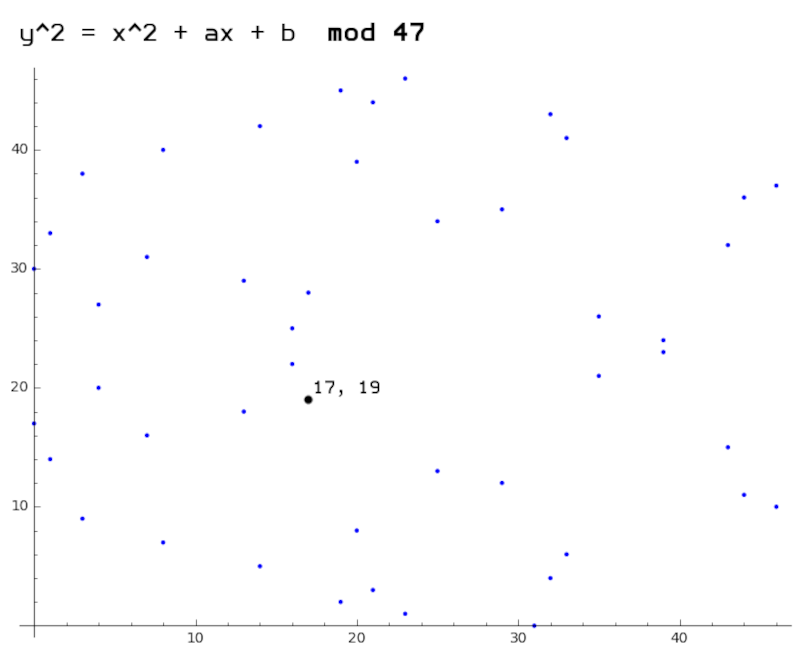Bitcoin's curve is over a finite set of numbers, we use mod to restrict ourselves within a certain range. This breaks a continuous curve that can be achieved using real numbers.

## Mathematics involved with Elliptic curves

In this section, we will be looking at different mathematical operations that can be performed on different points on the elliptic curve. The major two are double() and add() operations, occasionally, these are combined to perform a multiply() operation.

These operations are foundational to elliptic curve cryptography in the generation of public-private key pairs and digital signatures in ECDSA

#### Modular Inverse

For us to perform double and add operations, we should be able to calculate the modular inverse of a number in a finite field. We will apply a multiplication by an inverse within a finite field to achieve a result close to a division operation.

That is, if we start at a number in a finite field and multiply it by another number, we can reverse back to the number we began with by multiplying it again by the inverse of a number we used during multiplication.

The inverse function looks as shown below;

``````def mod_inv(k, p): # inverse k mod p
if k == 0:
raise ZeroDivisionError('division by zero')

if k < 0:
return p - mod_inv(-k, p)

# Extended Euclidean algorithm.
s, old_s = 0, 1
t, old_t = 1, 0
r, old_r = p, k

while r != 0:
quotient = old_r // r
old_r, r = r, old_r - quotient * r
old_s, s = s, old_s - quotient * s
old_t, t = t, old_t - quotient * t

gcd, x, y = old_r, old_s, old_t

assert gcd == 1
assert (k * x) % p == 1

return x % p
``````

Above we use the extended euclidean algorithm to calculate the modular inverse of a number. usually in mathematical equations modulus is denoted by a -1 as shown below;
${\mathrm{x}}^{-1}$

#### Double

Doubling involves adding a point to itself. For us to double a point we draw a tangent to the curve at a point and then find a point on the curve which the tangent intersects with. We then take the reflection of the point across the x-axis. This is demonstrated below;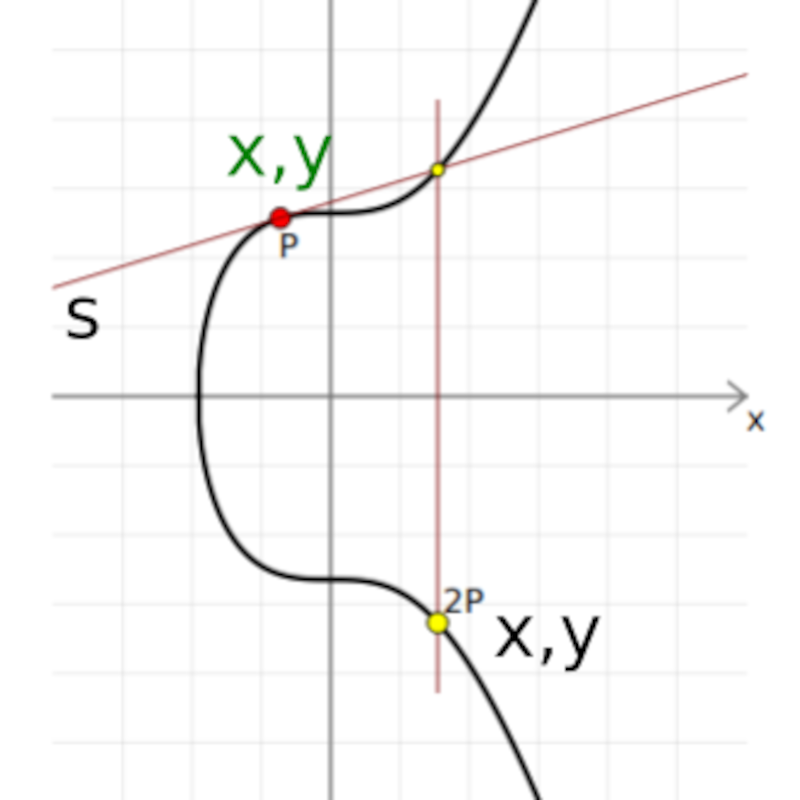Let's implement this in python;

``````def double(xp, yp):
num = 3 * xp * xp + a
deno = 2 * yp
l = (l * mod_inv(deno, prime)) % prime
xr = (l * l - 2 * xp) % prime
yr = (l * (xp - xr) - yp) % prime
return (xr, yr)
``````

Adding two points together on a curve involves drawing a line between them and finding a point on the curve where the line intersects with the curve. We then take the reflection of this point of intersection across the x-axis;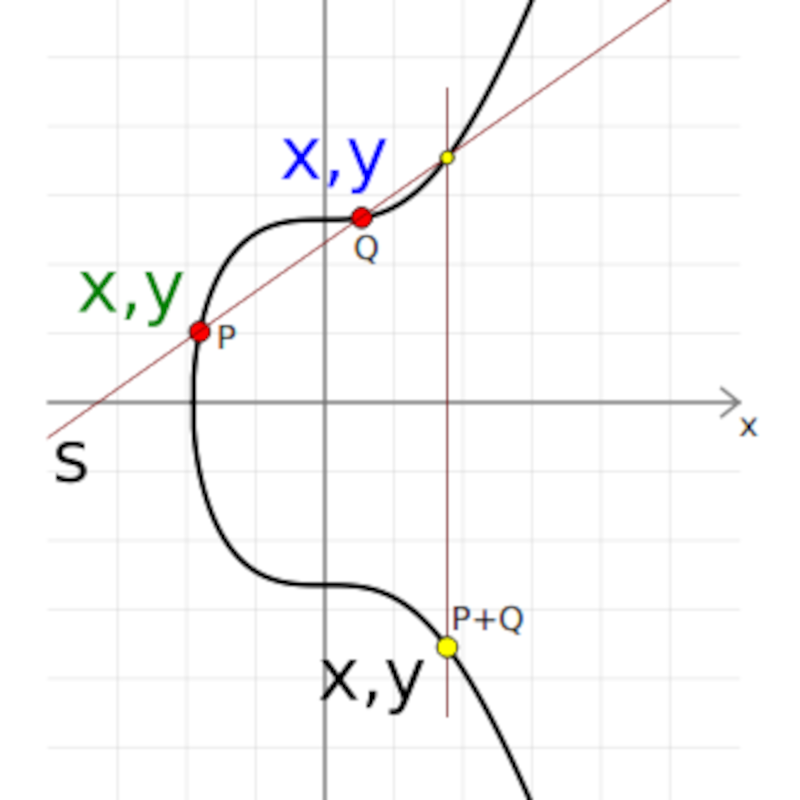The implementation in python is shown below;

``````def add(p1, p2): # addition operation
assert on_curve(p1) # check if points are on the curve
assert on_curve(p2)

if p1 is None:
return p2
if p2 is None:
return p1

x1, y1 = p1
x2, y2 = p2

if x1 == x2 and y1 != y2:
return None

if x1 == x2:
m = (3 * x1 * x1 + curve.a) * mod_inv(2 * y1, curve.p)
else:
m = (y1 - y2) * mod_inv(x1 - x2, curve.p)

x3 = m * m - x1 - x2
y3 = y1 + m * (x3 - x1)
result = (x3 % curve.p, -y3 % curve.p)

assert on_curve(result)

return result
``````

#### Multiply

Multiplication is very important in elliptic cryptography. A double-and-add algorithm is used. It implements both doubling and addition for it to reach the targeted multiple using the least number of operations.
For example, starting at 2 we can reach the target multiple 64 using five double() operations. This is shorter compared to using add() operations.

To determine the number of double and add operations needed to reach the target multiple we use the binary representation of an integer. For example;
Given the number 36 whose binary equivalent is 100100, for all 0 we use the double() operation, and for all 1 bits we perform the double and add() operation.
Therefore in oure example, we would, double_add(), double(), double(), double_add(), double(), double().

The python implementation is shown below;

``````def multiply(k, point): # multiplication function
assert on_curve(point)

if k % curve.n == 0 or point is None:
return None

if k < 0:
return multiply(-k, point_neg(point))

result = None

while k:
if k & 1:

k >>= 1

assert on_curve(result)

return result
``````

## Summary

The ECDSA algorithm is responsible for securing transactions and coins in Bitcoin and Ethereum blockchains.
An elliptic curve is a set of points described by the equation; y^2 = x^3 + ax + b mod p.
Two major operations double() and add() operations are foundational to elliptic curve cryptography in the generation of public-private key pairs and digital signatures in the ECDSA algorithm. Occasionally, these two are combined to perform a multiply() operation.

With this article at OpenGenus, you must have the complete idea of ECDSA Algorithm and Elliptic Curves.